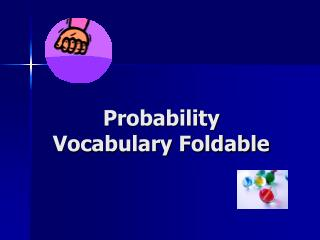DownloadDownload PresentationProbability Vocabulary Foldable

# Probability Vocabulary Foldable

Télécharger la présentation## Probability Vocabulary Foldable

- - - - - - - - - - - - - - - - - - - - - - - - - - - E N D - - - - - - - - - - - - - - - - - - - - - - - - - - -
##### Presentation Transcript

1. Probability Vocabulary Foldable

2. FOLD Simple Event Sample Space Probability Independent Events Relative FrequencyCompound Event Complement Addition Principle FOLD Fold edges to center and label outside like this

3. Sample Space Definition & Example The list of all possible outcomes for an activity. Pairs of Socks in Drawer 2 black 3 white 1 pink 1 green Activity – picking 1 pair of socks

4. Probability Definition & Example The chance an event will happen. Written as a number from 0 to 1. Impossible event - probability 0 Certain event – probability 1 Probability of event Z; P(Z) number of ways an event can occur number of possible outcomes P(black) = 2/7 P(white) = 3/7 P(green) = 1/7 P(orange) = 0 – no orange socks P(black, or white, or green, or pink) =7/7 or 1 or 100% Can be written as a decimal, fraction or percent

5. Relative Frequency Definition & Example The number of times an outcome occurs divided by the total number of trials or times attempted. Many trials – relative frequency = theoretical probability P(pink) = 1/7 I pick from the box 2 times and get pink both times. So… Relative frequency = 2/2 or 100%

6. Complement Definition & Example P(black) = 2/7 P(not black) or P(black’) = 1-(2/7) or 5/7 The opposite of an event happening

7. Simple Event Definition & Example Drawing one sock from the box. Only one pair/color is possible. An event with just one outcome possible at a time. Represented by one branch of a tree diagram.

8. Independent Events Definition & Example Drawing a pair of socks from the drawer and pulling out a pair of earrings from a jewelry box. Events that have no impact on how each other turn out.

9. Compound Event Definition & Example An event made up of 2 or more simple events. Picking a black sock , replacing it, AND picking a green sock. 2/7 * 1/7 = 2/49 MULTIPLY to get Probability of Compound Events One Event AND Another Event - MULTIPLY

10. Addition Principle Definition & Example If outcomes of 2 simple events are mutually exclusive (none the same), ADD their probability to find probability of both happening. Pick a pink sock OR pick a white sock. 1/7 + 3/7 = 4/7 One EventORAnother Event - ADD